Published in Comput. Meth. Appl. Mech. Engng., Vol. 197 (19-20), pp. 1777–1800, 2008
doi: 10.1016/j.cma.2007.06.005

## Abstract

We present some advances in the formulation of the Particle Finite Element Method (PFEM) for solving complex fluid-structure interaction problems with free surface waves. In particular, we present extensions of the PFEM for the analysis of the interaction between a collection of bodies in water allowing for frictional contact conditions at the fluid-solid and solid-solid interfaces via mesh generation. An algorithm to treat bed erosion in free surface flows is also presented. Examples of application of the PFEM to solve a number of fluid-multibody interaction problems involving splashing of waves, large motions of floating and submerged bodies and bed erosion situations are given.

Keywords: Lagrangian formulation, fluid-structure interaction, particle finite element method, bed erosion, free surface flows

## 1 INTRODUCTION

The analysis of problems involving the interaction of fluids and structures accounting for large motions of the fluid free surface and the existence of fully or partially submerged bodies which interact among themselves is of big relevance in many areas of engineering. Examples are common in ship hydrodynamics, off-shore and harbour structures, spill-ways in dams, free surface channel flows, environmental flows, liquid containers, stirring reactors, mould filling processes, etc.

Typical difficulties of fluid-multibody interaction analysis in free surface flows using the FEM with both the Eulerian and ALE formulation include the treatment of the convective terms and the incompressibility constraint in the fluid equations, the modelling and tracking of the free surface in the fluid, the transfer of information between the fluid and the moving solid domains via the contact interfaces, the modeling of wave splashing, the possibility to deal with large motions of the bodies within the fluid domain, the efficient updating of the finite element meshes for both the structure and the fluid, etc. For a comprehensive list of references in FEM for fluid flow problems see [5,34] and the references there included. A survey of recent works in fluid-structure interaction analysis can be found in ,  and .

Most of the above problems disappear if a Lagrangian description is used to formulate the governing equations of both the solid and the fluid domains. In the Lagrangian formulation the motion of the individual particles are followed and, consequently, nodes in a finite element mesh can be viewed as moving material points (hereforth called “particles”). Hence, the motion of the mesh discretizing the total domain (including both the fluid and solid parts) is followed during the transient solution.

The authors have successfully developed in previous works a particular class of Lagrangian formulation for solving problems involving complex interaction between fluids and solids. The method, called the particle finite element method (PFEM), treats the mesh nodes in the fluid and solid domains as particles which can freely move and even separate from the main fluid domain representing, for instance, the effect of water drops. A finite element mesh connects the nodes defining the discretized domain where the governing equations are solved using a stabilized FEM based in the Finite Calculus (FIC) approach. An advantage of the Lagrangian formulation is that the convective terms disappear from the fluid equations. The difficulty is however transferred to the problem of adequately (and efficiently) moving the mesh nodes. We use a mesh regeneration procedure blending elements of different shapes using an extended Delaunay tesselation with special shape functions [9,11]. The theory and applications of the PFEM are reported in [2,4,9,10,12,13,24,25,26,28,29].

The aim of this paper is to describe two recent advances of the PFEM: a) the analysis of the interaction between a collection of bodies which are floating and/or submerged in the fluid, and b) the modeling of bed erosion in open channel flows. Both problems are of great relevance in many areas of civil, marine and naval engineering, among others. It is shown in the paper that the PFEM provides a general analysis methodology for treat such a complex problems in a simple and efficient manner.

The layout of the paper is the following. In the next section the key ideas of the PFEM are outlined. Next the basic equations for an incompressible flow using a Lagrangian description and the FIC formulation are presented. Then a fractional step scheme for the transient solution is briefly described. Details of the treatment of the coupled FSI problem are given. The methods for mesh generation and for identification of the free surface nodes are outlined. The procedure for treating at mesh generation level the contact conditions at fluid-wall interfaces and the frictional contact interaction between moving solids is explained. A methodology for modeling bed erosion due to fluid forces is described. Finally, the efficiency of the PFEM is shown in its application to a number of problems involving large flow motions, surface waves, moving bodies in water and bed erosion.

## 2 THE BASIS OF THE PARTICLE FINITE ELEMENT METHOD

Let us consider a domain containing both fluid and solid subdomains. The moving fluid particles interact with the solid boundaries thereby inducing the deformation of the solid which in turn affects the flow motion and, therefore, the problem is fully coupled.

In the PFEM both the fluid and the solid domains are modelled using an updated Lagrangian formulation. That is, all variables in the fluid and solid domains are assumed to be known in the current configuration at time ${\textstyle t}$. The new set of variables in both domains are sought for in the next or updated configuration at time ${\textstyle t+\Delta t}$ (Figure 1). The finite element method (FEM) is used to solve the continuum equations in both domains. Hence a mesh discretizing these domains must be generated in order to solve the governing equations for both the fluid and solid problems in the standard FEM fashion. Recall that the nodes discretizing the fluid and solid domains are treated as material particles which motion is tracked during the transient solution. This is useful to model the separation of fluid particles from the main fluid domain in a splashing wave, or soil particles in a bed erosion problem, and to follow their subsequent motion as individual particles with a known density, an initial acceleration and velocity and subject to gravity forces. The mass of a given domain is obtained by integrating the density at the different material points over the domain.

The quality of the numerical solution depends on the discretization chosen as in the standard FEM. Adaptive mesh refinement techniques can be used to improve the solution in zones where large motions of the fluid or the structure occur.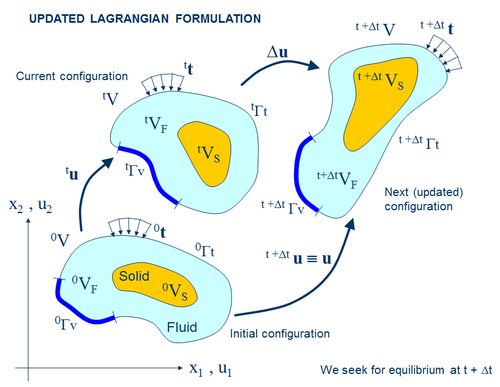Figure 1: Updated lagrangian description for a continuum containing a fluid and a solid domain

### 2.1 Basic steps of the PFEM

For clarity purposes we will define the collection or cloud of nodes (C) pertaining to the fluid and solid domains, the volume (V) defining the analysis domain for the fluid and the solid and the mesh (M) discretizing both domains.

A typical solution with the PFEM involves the following steps.

1. The starting point at each time step is the cloud of points in the fluid and solid domains. For instance ${\textstyle {}^{n}C}$ denotes the cloud at time ${\textstyle t=t_{n}}$ (Figure 2).
2. Identify the boundaries for both the fluid and solid domains defining the analysis domain ${\textstyle {}^{n}V}$ in the fluid and the solid. This is an essential step as some boundaries (such as the free surface in fluids) may be severely distorted during the solution, including separation and re-entering of nodes. The Alpha Shape method  is used for the boundary definition (Section 5).
3. Discretize the fluid and solid domains with a finite element mesh ${\textstyle {}^{n}M}$. In our work we use an innovative mesh generation scheme based on the extended Delaunay tesselation (Section 4) [9,10,12].
4. Solve the coupled Lagrangian equations of motion for the fluid and the solid domains. Compute the relevant state variables in both domains at the next (updated) configuration for ${\textstyle t+\Delta t}$: velocities, pressure and viscous stresses in the fluid and displacements, stresses and strains in the solid.
5. Move the mesh nodes to a new position ${\textstyle {}^{n+1}C}$ where ${\textstyle n+1}$ denotes the time ${\textstyle t_{n}+\Delta t}$, in terms of the time increment size. This step is typically a consequence of the solution process of step 4.
6. Go back to step 1 and repeat the solution process for the next time step to obtain ${\textstyle {}^{n+2}C}$. The process is shown in Figure 2.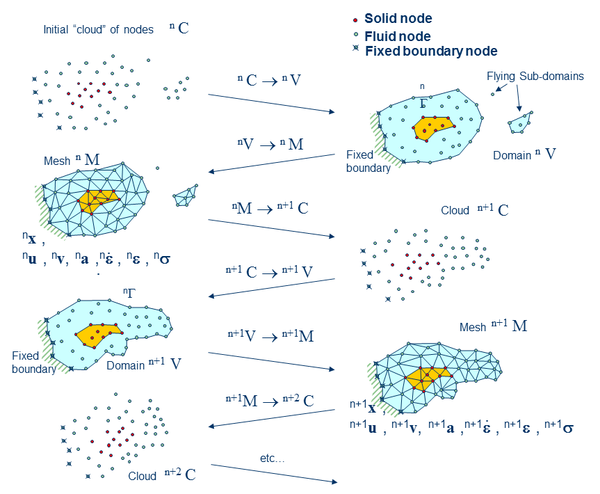Figure 2: Sequence of steps to update a “cloud” of nodes from time $n$ ($t=t_{n}$) to time $n+2$ ($t=t_{n}+2\Delta t$)

### 2.2 Overview of the coupled FSI algoritm

Figure 3 shows a typical domain ${\textstyle V}$ with external boundaries ${\textstyle \Gamma _{V}}$ and ${\textstyle \Gamma _{t}}$ where the velocity and the surface tractions are prescribed, respectively. The domain ${\textstyle V}$ is formed by fluid (${\textstyle V_{F}}$) and solid (${\textstyle V_{S}}$) subdomains (i.e. ${\textstyle V=V_{F}\cup V_{S}}$). Both subdomains interact at a common boundary ${\textstyle \Gamma _{FS}}$ where the surface tractions and the kinematic variables (displacements, velocities and acelerations) are the same for both subdomains. Note that both set of variables (the surface tractions and the kinematic variables) are equivalent in the equilibrium configuration.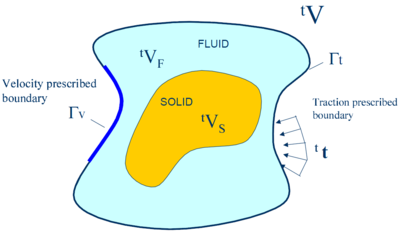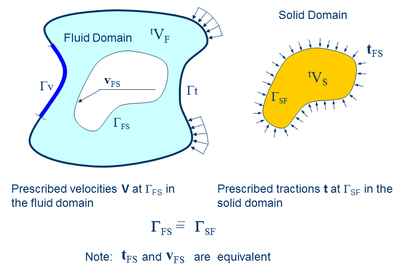Figure 3: Split of the analysis domain $V$ into fluid and solid subdomains. Equality of surface tractions and kinematic variables at the common interface

Let us define ${\textstyle {}^{t}S}$ and ${\textstyle {}^{t}F}$ the set of variables defining the kinematics and the stress-strain fields at the solid and fluid domains at time ${\textstyle t}$, respectively, i.e.

 ${}^{t}S:=[{}^{t}{x}_{s},{}^{t}{u}_{s},{}^{t}{v}_{s},{}^{t}{a}_{s},{}^{t}{\boldsymbol {\varepsilon }}_{s},{}^{t}{\boldsymbol {\sigma }}_{s},\cdots ]^{T}$ (1) ${}^{t}F:=[{}^{t}{x}_{F},{}^{t}{u}_{F},{}^{t}{v}_{F},{}^{t}{a}_{F},{}^{t}{\dot {\boldsymbol {\varepsilon }}}_{F},{}^{t}{\boldsymbol {\sigma }}_{F},\cdots ]^{T}$ (2)

where ${\textstyle {x}}$ is the nodal coordinate vector, u, v and a are the vector of displacements, velocities and accelerations, respectively, ${\textstyle {\boldsymbol {\varepsilon }},{\dot {\boldsymbol {\varepsilon }}}}$ and ${\textstyle {\boldsymbol {\sigma }}}$ are the strain vector, the strain-rate (or rate of deformation) vectors and the Cauchy stress vector, respectively and ${\textstyle F}$ and ${\textstyle S}$ denote the variables in the fluid and solid domains, respectively. In the discretized problem, a bar over these variables will denote nodal values.

The coupled fluid-structure interaction (FSI) problem of Figure 3 is solved, in this work, using the following strongly coupled staggered scheme:

1. We assume that the variables in the solid and fluid domains at time ${\textstyle t}$ (${\textstyle {}^{t}S}$ and ${\textstyle {}^{t}F}$) are known.
2. Solve for the variables at the solid domain at time ${\textstyle t+\Delta t}$ (${\textstyle {}^{t+\Delta t}{S}}$) under prescribed surface tractions at the fluid-solid boundary ${\textstyle \Gamma _{FS}}$. The boundary conditions at the part of the external boundary intersecting the domain are the standard ones in solid mechanics.
3. Solve for the variables at the fluid domain at time ${\textstyle t+\Delta t}$ (${\textstyle {}^{t+\Delta t}{F}}$) under prescribed surface tractions at the external boundary ${\textstyle \Gamma _{t}}$ and prescribed velocities at the external and internal boundaries ${\textstyle \Gamma _{V}}$ and ${\textstyle \Gamma _{FS}}$, respectively.
4. Iterate between 1 and 2 until convergence.

The variables at the solid domain ${\textstyle {}^{t+\Delta t}{S}}$ are found via the integration of the equations of dynamic motion in the solid written as

 ${M}_{s}{a}_{s}+{g}_{s}-{f}_{s}={0}$
(3)

where ${\textstyle {M}_{s},{g}_{s}}$ and ${\textstyle {f}_{s}}$ denote the mass matrix, the internal node force vector and the external nodal force vector at the solid domain. The time integration of Eq.(3) is performed using a standard Newmark method. An incremental iterative scheme is implemented within each time step to account for non linear geometrical and material effects.

The FEM solution of the variables in the (incompressible) fluid domain implies solving the momentum and incompressibility equations. As mentioned above this is not such as simple problem as the incompressibility condition limits the choice of the FE approximations for the velocity and pressure to overcome the well known ${\textstyle div}$-stability condition [5,34]. In our work we use a stabilized mixed FEM based on the Finite Calculus (FIC) approach which allows for a linear approximation for the velocity and pressure variables. Details of the FEM/FIC formulation are given in the next section.

Figure 4 shows a typical example of a PFEM solution in 2D. The pictures correspond to the analysis of the problem of breakage of a water column [12,26]. Figure 4a shows the initial grid of four node rectangles discretizing the fluid domain and the solid walls. Figures 4b and 4c show the mesh for the solution at two later times.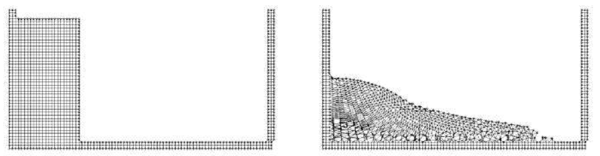Figure 4: Breakage of a water column. (a) Discretization of the fluid domain and the solid walls. Boundary nodes are marked with circles. (b) and (c) Mesh in the fluid domain at two different times.

## 3 FIC/FEM FORMULATION FOR A LAGRANGIAN INCOMPRESSIBLE FLUID

The standard infinitesimal equations for a viscous incompressible fluid can be written in a Lagrangian frame as [17,34].

Momentum

 $r_{m_{i}}=0\quad {\hbox{in }}V_{F}$
(4)

Mass balance

 $r_{d}=0\quad {\hbox{in }}V_{F}$
(5)

where

 $r_{m_{i}}=\rho {\partial v_{i} \over \partial t}+{\partial \sigma _{ij} \over \partial x_{j}}-b_{i}\quad ,\quad \sigma _{ji}=\sigma _{ij}$ (6) $r_{d}={\partial v_{i} \over \partial x_{i}}\qquad i,j=1,n_{d}$ (7)

Above ${\textstyle n_{d}}$ is the number of space dimensions, ${\textstyle v_{i}}$ is the velocity along the ith global axis (${\textstyle v_{i}={\partial u_{i} \over \partial t}}$, where ${\textstyle u_{i}}$ is the ith displacement), ${\textstyle \rho }$ is the (constant) density of the fluid, ${\textstyle b_{i}}$ are the body forces, ${\textstyle \sigma _{ij}}$ are the total stresses given by ${\textstyle \sigma _{ij}=s_{ij}-\delta _{ij}p}$, ${\textstyle p}$ is the absolute pressure (defined positive in compression) and ${\textstyle s_{ij}}$ are the viscous deviatoric stresses related to the viscosity ${\textstyle \mu }$ by the standard expression

 $s_{ij}=2\mu \left({\dot {\varepsilon }}_{ij}-\delta _{ij}{1 \over 3}{\partial v_{k} \over \partial x_{k}}\right)$
(8)

where ${\textstyle \delta _{ij}}$ is the Kronecker delta and the strain rates ${\textstyle {\dot {\varepsilon }}_{ij}}$ are

 ${\dot {\varepsilon }}_{ij}={1 \over 2}\left({\partial v_{i} \over \partial x_{j}}+{\partial v_{j} \over \partial x_{i}}\right)$
(9)

In the above all variables are defined at the current time ${\textstyle t}$ (current configuration).

In our work we will solve a modified set of governing equations derived using a finite calculus (FIC) formulation. The FIC governing equations are [17,18,19,21].

Momentum

 $r_{m_{i}}-{\underline {{1 \over 2}h_{j}{\partial r_{m_{i}} \over \partial x_{j}}}}{1 \over 2}h_{j}{\partial r_{m_{i}} \over \partial x_{j}}=0\qquad {\hbox{in }}V_{F}$
(10)

Mass balance

 $r_{d}-{\underline {{1 \over 2}h_{j}{\partial r_{d} \over \partial x_{j}}}}{1 \over 2}h_{j}{\partial r_{d} \over \partial x_{j}}=0\qquad {\hbox{in }}V_{F}$
(11)

The problem definition is completed with the following boundary conditions

 $n_{j}\sigma _{ij}-t_{i}+{\underline {{1 \over 2}h_{j}n_{j}r_{m_{i}}}}{1 \over 2}h_{j}n_{j}r_{m_{i}}=0\quad {\hbox{on }}\Gamma _{t}$
(12)
 $v_{j}-v_{j}^{p}=0\quad {\hbox{on }}\Gamma _{v}$
(13)

and the initial condition is ${\textstyle v_{j}=v_{j}^{0}}$ for ${\textstyle t=t_{0}}$. The standard summation convention for repeated indexes is assumed unless otherwise specified.

In Eqs.(12) and (13) ${\textstyle t_{i}}$ and ${\textstyle v_{j}^{p}}$ are surface tractions and prescribed velocities on the boundaries ${\textstyle \Gamma _{t}}$ and ${\textstyle \Gamma _{v}}$, respectively, ${\textstyle n_{j}}$ are the components of the unit normal vector to the boundary. Recall that ${\textstyle \Gamma _{v}}$ includes both the external boundary and the internal boundary ${\textstyle \Gamma _{F_{S}}}$ (Figure 3).

The ${\textstyle h_{i}'s}$ in above equations are characteristic lengths of the domain where balance of momentum and mass is enforced. In Eq.(12) these lengths define the domain where equilibrium of boundary tractions is established. We note that at the discretized level, the ${\textstyle h_{i}'s}$ become of the order of a typical element or grid dimension [17,18,19].

Eqs.(10)–(13) are the starting point for deriving stabilized finite element methods to solve the incompressible Navier-Stokes equations in a Lagrangian frame of reference using equal order interpolation for the velocity and pressure variables [2,8,9,10,12,24]. Application of the FIC formulation to finite element and meshless analysis of fluid flow problems can be found in [7,18,19,20,21,23,25,27].

### 3.1 Transformation of the mass balance equation. Integral governing equations

The underlined term in Eq.(11) can be expressed in terms of the momentum equations. The new expression for the mass balance equation is [18,26]

 $r_{d}-\sum \limits _{i=1}^{n_{d}}\tau _{i}{\partial r_{m_{i}} \over \partial x_{i}}=0$
(14)

with

 $\tau _{i}={3h_{i}^{2} \over 8\mu }$
(15)

In our work we have taken the characteristic distances ${\textstyle h_{i}}$ to be constant within each element and equal to a typical element dimension computed as ${\textstyle h^{e}=[V^{e}]^{m}}$ where ${\textstyle V^{e}}$ is the element domain and ${\textstyle m=1/2}$ for 2D problems and ${\textstyle m=1/3}$ for 3D problems.

At this stage it is no longer necessary to retain the stabilization terms in the momentum equations and the traction boundary conditions (Eqs.(10) and (12)). These terms are critical in Eulerian formulations to stabilize the numerical solution for high values of the convective terms [18,21,27,28].

The weighted residual expression of the final form of the momentum and mass balance equations is written as

 $\int _{V_{F}}\delta v_{i}r_{m_{i}}dV+\int _{\Gamma _{t}}\delta v_{i}(n_{j}\sigma _{ij}-t_{i})d\Gamma =0$
(16)
 $\int _{V_{F}}q\left[r_{d}-\sum \limits _{i=1}^{n_{d}}\tau _{i}{\partial r_{m_{i}} \over \partial x_{i}}\right]dV=0$
(17)

where ${\textstyle \delta v_{i}}$ and ${\textstyle q}$ are arbitrary weighting functions equivalent to virtual velocity and virtual pressure fields.

The ${\textstyle r_{m_{i}}}$ term in Eq.(17) and the deviatoric stresses and the pressure terms within ${\textstyle r_{m_{i}}}$ in Eq.(16) are integrated by parts to give

 $\int _{V_{F}}\left[\delta v_{i}\rho {\partial v_{i} \over \partial t}+\delta {\dot {\varepsilon }}_{ij}(s_{ij}-\delta _{ij}p)\right]dV-\int _{V_{F}}\delta v_{i}b_{i}d\Omega -\int _{\Gamma _{t}}\delta v_{i}t_{i}d\Gamma =0$
(18)
 $\int _{V_{F}}q{\partial v_{i} \over \partial x_{i}}dV+\int _{V_{F}}\left[\sum \limits _{i=1}^{n_{d}}\tau _{i}{\partial q \over \partial x_{i}}r_{m_{i}}\right]dV=0$
(19)

In Eq.(18) ${\textstyle \delta {\dot {\varepsilon }}_{ij}}$ are virtual strain rates. Note that the boundary term resulting from the integration by parts of ${\textstyle r_{m_{i}}}$ in Eq.(19) has been neglected in this work. Retaining this term has been recently found to be advantageous for enhancing the satisfaction of the incompressibility condition in FEM predictor-corrector schemes for incompressible fluid flow analysis .

The computation of the residual terms in Eq.(19) is simplified if we introduce the pressure gradient projections ${\textstyle \pi _{i}}$, defined as

 $\pi _{i}=r_{m_{i}}-{\partial p \over \partial x_{i}}$
(20)

We express ${\textstyle r_{m_{i}}}$ in Eq.(19) in terms of the ${\textstyle \pi _{i}}$ which then become additional variables. The system of integral equations is now augmented in the necessary number of equations by imposing that the residual ${\textstyle r_{m_{i}}}$ vanishes within the analysis domain (in an average sense). This gives the final system of governing equation as:

 $\int _{V_{F}}\left[\delta v_{i}\rho {\partial v_{i} \over \partial t}+\delta {\dot {\varepsilon }}_{ij}(s_{ij}-\delta _{ij}p)\right]dV-\int _{V_{F}}\delta v_{i}b_{i}dV-\int _{\Gamma _{t}}\delta v_{i}t_{i}d\Gamma =0$
(21)
 $\int _{V_{F}}q{\partial v_{i} \over \partial x_{i}}dV+\int _{V_{F}}\sum \limits _{i=1}^{n_{d}}\tau _{i}{\partial q \over \partial x_{i}}\left({\partial p \over \partial x_{i}}+\pi _{i}\right)dV=0$
(22)
 $\int _{V_{F}}\delta \pi _{i}\tau _{i}\left({\partial p \over \partial x_{i}}+\pi _{i}\right)dV=0\qquad {\hbox{no sum in }}i$
(23)

with ${\textstyle i,j,k=1,n_{d}}$. In Eqs.(23) ${\textstyle \delta \pi _{i}}$ are appropriate weighting functions and the ${\textstyle \tau _{i}}$ weights are introduced for symmetry reasons.

### 3.3 Finite element discretization

We choose equal order ${\textstyle C^{\circ }}$ continuous interpolations of the velocities, the pressure and the pressure gradient projections ${\textstyle \pi _{i}}$ over each element with ${\textstyle n}$ nodes. The interpolations are written as

 $v_{i}=\sum \limits _{j=1}^{n}N_{j}{\bar {v}}_{i}^{j}\quad ,\quad p=\sum \limits _{j=1}^{n}N_{j}{\bar {p}}^{j}\quad ,\quad \pi _{i}=\sum \limits _{j=1}^{n}N_{j}{\bar {\pi }}_{i}^{j}$
(24)

where ${\textstyle {\bar {(\cdot )}}^{j}}$ denotes nodal variables and ${\textstyle N_{j}}$ are the shape functions . More details of the mesh discretization process and the choice of shape functions are given in Section 4.

Substituting the approximations (24) into Eqs.(21–23) and choosing a Galerkin form with ${\textstyle \delta v_{i}=q=\delta \pi _{i}=N_{i}}$ leads to the following system of discretized equations

 ${M}{\dot {\bar {v}}}+{K}{\bar {v}}-{G}{\bar {p}}-{f}={0}$
(25.a)
 $\displaystyle {G}^{T}{\bar {v}}+{L}{\bar {p}}+{Q}{\bar {\boldsymbol {\pi }}}={0}$
(25.b)
 $\displaystyle {Q}^{T}{\bar {p}}+{\hat {M}}{\bar {\boldsymbol {\pi }}}={0}$
(25.c)

The form of the element matrices and vectors in Eqs.(25) can be found in .

### 3.4 Fractional step algorithm for the fluid variables

The starting point of the iterative algorithm are the variables at time ${\textstyle n}$ in the fluid domain (${\textstyle {}^{n}F}$). The sought variables are the variables at time ${\textstyle n+1}$ (${\textstyle {}^{n+1}F}$). For the sake of clarity we will skip the upper left index ${\textstyle n+1}$ for all variables, i.e.

 ${}^{n+1}{\bar {x}}\equiv {\bar {x}}\,\,;\,\,{}^{n+1}{\bar {p}}\equiv {\bar {p}}\,\,;\,\,{}^{n+1}{\bar {\boldsymbol {\pi }}}\equiv {\bar {\boldsymbol {\pi }}}\,\,;\,\,{}^{n+1}{\bar {x}}\equiv {\bar {x}}\,\,;{\hbox{etc.}}$
(26)

A simple iterative algorithm is obtained by splitting the pressure from the momentum equations as follows

 ${\bar {v}}^{*}={}^{n}{\bar {v}}-\Delta t{M}^{-1}[{K}{\bar {v}}^{j}-{G}{}^{n}\mathbf {p} -{f}]$
(27)
 ${\bar {v}}^{j+1}={\bar {v}}^{*}+\Delta t{M}^{-1}{G}\delta {\bar {p}}$
(28)

where ${\textstyle \delta {\bar {p}}}$ denotes the pressure increment. In above equations and in the following the left upper index ${\textstyle n}$ refers to values in the current configuration ${\textstyle {}^{n}V}$, whereas the right upper index ${\textstyle j}$ denotes the iteration number within each time step.

The value of ${\textstyle {\bar {v}}^{j+1}}$ from Eq.(29) is substituted now into Eq.(25b) to give

 ${G}^{T}{\bar {v}}^{*}+\Delta t{S}\delta {\bar {p}}+{L}{\bar {p}}^{j+1}+{Q}{\bar {\boldsymbol {\pi }}}^{j}={0}$
(29.a)

where

 ${S}={G}^{T}{M}^{-1}{G}$
(29.b)

Typically matrix S is computed using a diagonal matrix ${\textstyle {M}={M}_{d}}$, where the subscript ${\textstyle d}$ denotes hereonward a diagonal matrix. We note that the form of S in Eq.(29b) avoids the need for prescribing the pressure at the boundary nodes.

An alternative is to approximate S by a Laplacian matrix. This reduces considerably the bandwidth of S. The disadvantage is that the pressure increment must be then prescribed on the free surface and this reduces the accuracy in the satisfaction of the incompressibility condition in these regions. These problems are overcome however by retaining the residual term ${\textstyle r_{m_{i}}}$ in the boundary integral resulting from the integration by parts of Eq.(17) . In this work however the form of matrix S given by Eq.(29a) has been used.

A semi-implicit algorithm can be derived as follows. For each iteration:

Step 1 Compute ${\textstyle {v}^{*}}$ from Eq.(27) with ${\textstyle {M}={M}_{d}}$. For the first iteration

${\textstyle ({\bar {v}}^{1},{\bar {p}}^{1},{\bar {\boldsymbol {\pi }}}^{1},{\bar {x}}^{1})\equiv ({}^{n}{\bar {v}},{}^{n}{\bar {p}},{}^{n}{\bar {\boldsymbol {\pi }}},{}^{n}{\bar {x}})}$

Step 2 Compute ${\textstyle \delta {\bar {p}}}$ and ${\textstyle {p}^{j+1}}$ from Eq.(29a) as

 $\delta {\bar {p}}=-({L}+\Delta t{S})^{-1}[{G}^{T}{\bar {v}}^{*}+{Q}{\bar {\boldsymbol {\pi }}}^{j}+{L}{\bar {p}}^{j}]$
(30.a)

The pressure ${\textstyle {\bar {p}}^{n+1,j}}$ is computed as

 ${\bar {p}}^{j+1}={\bar {p}}^{j}+\delta {\bar {p}}^{j}$
(30.b)

Step 3 Compute ${\textstyle {\bar {v}}^{j+1}}$ from Eq.(28) with ${\textstyle {M}={M}_{d}}$

Step 4 Compute ${\textstyle {\bar {\boldsymbol {\pi }}}^{j+1}}$ from Eq.(25c) as

 ${\bar {\boldsymbol {\pi }}}^{j+1}=-{\hat {M}}_{d}^{-1}{Q}^{T}{\bar {p}}^{j+1}$
(31)

Step 5 Update the coordinates of the mesh nodes as

 ${x}_{i}^{j+1}={}^{n}{x}_{i}+{\bar {v}}_{i}^{j+1}\Delta t$
(32)

Step 6 Check the convergence of the velocity and pressure fields. If convergence is achieved move to the next time step, otherwise return to step 1 for the next iteration with ${\textstyle j\leftarrow j+1}$.

Note that solution of steps 1, 3 and 4 does not require the solution of a system of equations as a diagonal form is chosen for ${\textstyle M}$ and ${\textstyle {\hat {M}}}$.

In the examples presented in the paper the time increment size has been chosen as

 $\Delta t=\min(\Delta t_{i})\quad {\hbox{with}}\quad \Delta t_{i}={h_{i}^{\min } \over \vert {v}\vert }$
(33)

where ${\textstyle h_{i}^{\min }}$ is the distance between node ${\textstyle i}$ and the closest node in the mesh.

Although not explicitely mentioned all matrices and vectors in Eqs.(25) are computed at the updated configuration ${\textstyle {}^{n+1}V_{F}}$. This means that the integration domain changes for each iteration and, hence, all the terms involving space derivatives must be updated at each iteration.

The boundary conditions are applied as follows. No condition is applied for the computation of the fractional velocities ${\textstyle {v}^{*}}$ in Eq.(27). The prescribed velocities at the boundary are applied when solving for ${\textstyle {\bar {v}}^{j+1}}$ in step 3. As mentioned earlier there is no need for prescribing the pressure at the boundary if the form of Eq.(29b) is chosen for S.

Box 1 shows a summary of the staggered scheme used for the solution for the variables in the solid and fluid domain at the updated configuration (${\textstyle {}^{n+1}F,{}^{n+1}S}$).

Box 1. Staggered scheme for the FSI problem (see also Figure 3)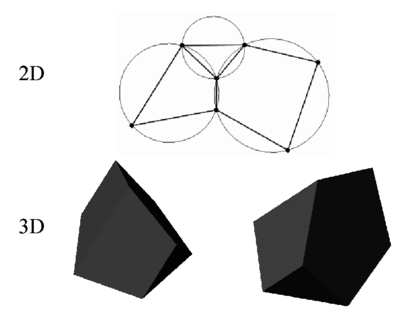Figure 5: Generation of non standard meshes combining different polygons (in 2D) and polyhedra (in 3D) using the extended Delaunay technique.

## 4 GENERATION OF A NEW MESH

One of the key points for the success of the PFEM is the fast regeneration of a mesh at every time step on the basis of the position of the nodes in the space domain. Indeed, any fast meshing algorithm can be used for this purpose. In our work the mesh is generated at each time step using the so called extended Delaunay tesselation (EDT) presented in [9,11,12]. The EDT allows one to generate non standard meshes combining elements of arbitrary polyhedrical shapes (triangles, quadrilaterals and other polygons in 2D and tetrahedra, hexahedra and arbitrary polyhedra in 3D) in a computing time of order ${\textstyle n}$, where ${\textstyle n}$ is the total number of nodes in the mesh (Figure 5). The ${\textstyle C^{\circ }}$ continuous shape functions of the elements can be simply obtained using the so called meshless finite element interpolation (MFEM). In our work the simpler linear C${\textstyle ^{\circ }}$ interpolation has been chosen. Details of the mesh generation procedure and the derivation of the linear MFEM shape functions can be found in [9,11,12].

Figure 6 shows the evolution of the CPU time required for generating the mesh, for solving the system of equations and for assembling such a system in terms of the number of nodes. the numbers correspond to the solution of a 3D flow in an open channel with the PFEM. The figure shows the CPU time in seconds for each time step of the algorithm of Section 3.4. It is clearly seen that the CPU time required for meshing grows linearly with the number of nodes, as expected. Note also that the CPU time for solving the equations exceeds that required for meshing as the number of nodes increases. This situation has been found in all the problems solved with the PFEM. As a general rule for large 3D problems meshing consumes around 30% of the total CPU time for each time step, while the solution of the equations and the assembling of the system consume approximately 40% and 20% of the CPU time for each time step, respectively. These figures prove that the generation of the mesh has an acceptable cost in the PFEM solution. An improvement of the mesh generation process will in any case help to reducing the computational cost.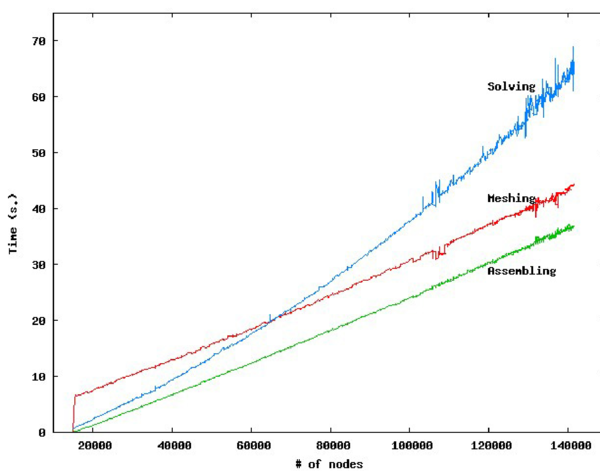Figure 6: 3D flow problem solved with the PFEM. CPU time for meshing, assembling and solving the system of equations at each time step in terms of the number of nodes

## 5 IDENTIFICATION OF BOUNDARY SURFACES

One of the main tasks in the PFEM is the correct definition of the boundary domain. Boundary nodes are sometimes explicitly identified. In other cases, the total set of nodes is the only information available and the algorithm must recognize the boundary nodes.

In our work we use an extended Delaunay partition for recognizing boundary nodes. Considering that the nodes follow a variable ${\textstyle h(x)}$ distribution, where ${\textstyle h(x)}$ is typically the minimum distance between two nodes, the following criterion has been used. All nodes on an empty sphere with a radius greater than $\alpha h$, are considered as boundary nodes. In practice ${\textstyle \alpha }$ is a parameter close to, but greater than one. Values of ${\textstyle \alpha }$ ranging between 1.3 and 1.5 have been found to be optimal in all examples analyzed. This criterion is coincident with the Alpha Shape concept . Figure 7 shows an example of the boundary recognition using the Alpha Shape technique.

Once a decision has been made concerning which nodes are on the boundaries, the boundary surface is defined by all the polyhedral surfaces (or polygons in 2D) having all their nodes on the boundary and belonging to just one polyhedron.

The method described also allows one to identify isolated fluid particles outside the main fluid domain. These particles are treated as part of the external boundary where the pressure is fixed to the atmospheric value. We recall that each particle is a material point characterized by the density of the solid or fluid domain to which it belongs. The mass which is lost when a boundary element is eliminated due to departure of a node (a particle) from the main analysis domain is again regained when the “flying” node falls down and a new boundary element is created by the Alpha Shape algorithm (Figures 2 and 7).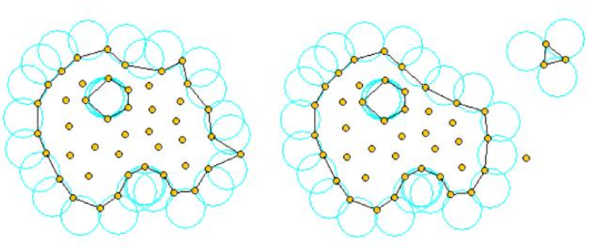Figure 7: Identification of individual particles (or a group of particles) starting from a given collection of nodes.

The boundary recognition method above described is also useful for detecting contact conditions between the fluid domain and a fixed boundary, as well as between different solids interacting with each other. The contact detection procedure is detailed in Section 6.

In order to show the quality of the boundary recognition approach, the following simple example has been performed. A square fluid domain of 0.25m${\textstyle ^{2}}$ is at a stationary position within a recipient (Figure 8). Then, as time evolves, the fluid falls down into the lower part of the recipient due to gravity effects. At the end of the process the total volume of the fluid within the recipient must be the same as that of the initial square domain. It must be noted that during the different time steps, the fluid has completely different free-surfaces including waves, breaking waves and fluid fragmentation zones.

The meshes used have average element sizes of 0.05m, 0.025m and 0.01m each which correspond to a total initial number of particles of 161, 552 and 3105 each. A value of ${\textstyle \alpha =1.4}$ for the Alpha-Shape method was used for the three analyses. Figure 8 shows the initial position of the fluid domain and one of the three meshes used for the analysis. Figure 9 shows the fluid domain at different time steps.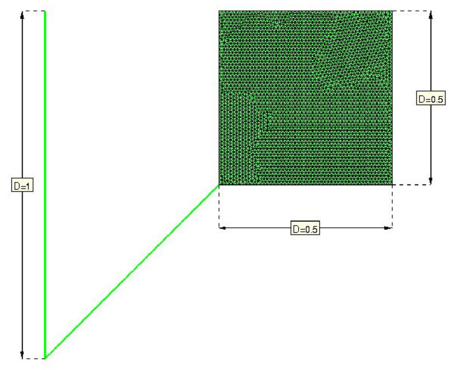Figure 8: Fluid domain following into a recipient. Initial position. Fine mesh of 3105 nodes (element size of 0.01m)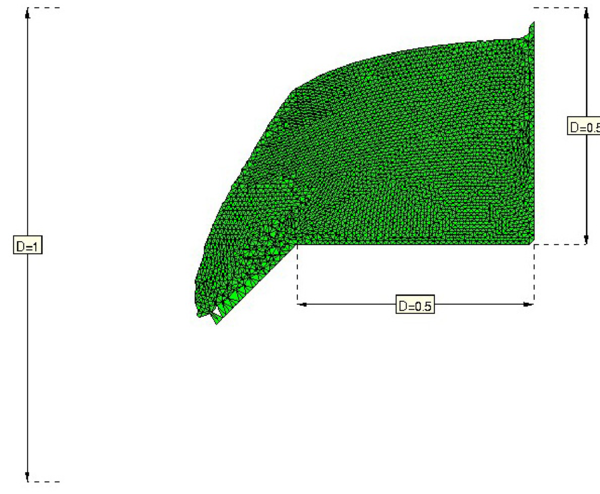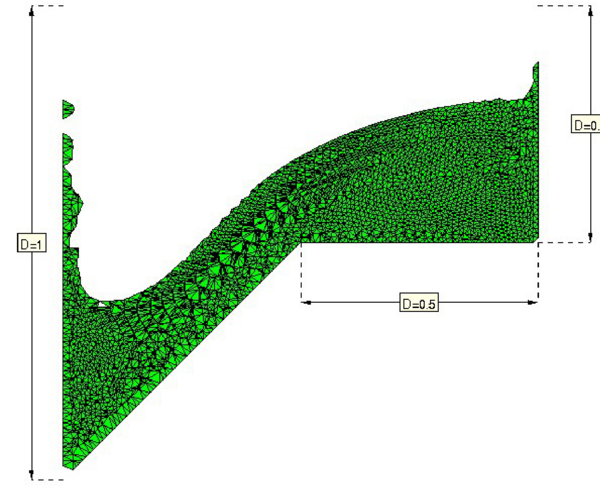(a) $t=0.2$s (b) $t=0.4$s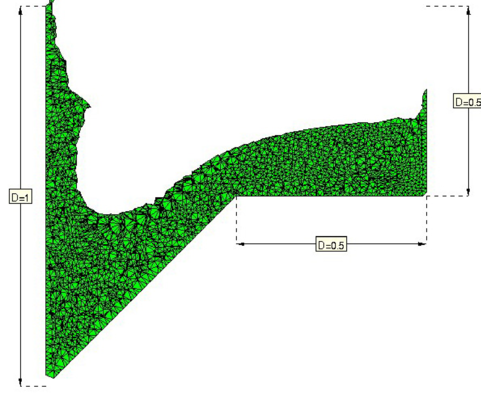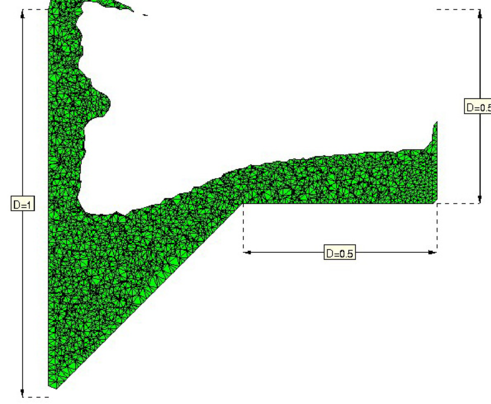(c) $t=0.6$s (d) $t=0.8$s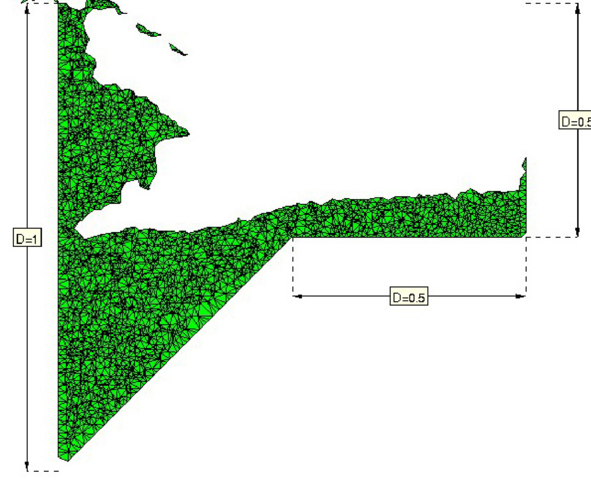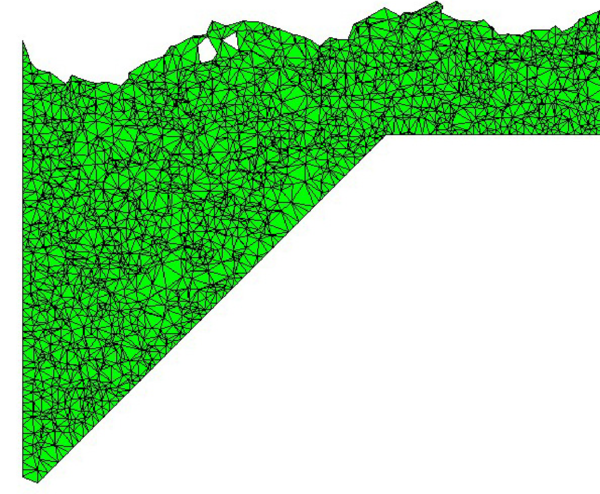(e) $t=1.0$s (f) $t=2.0$s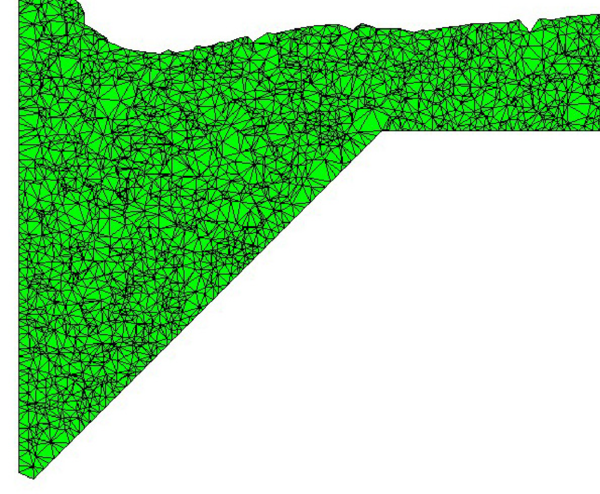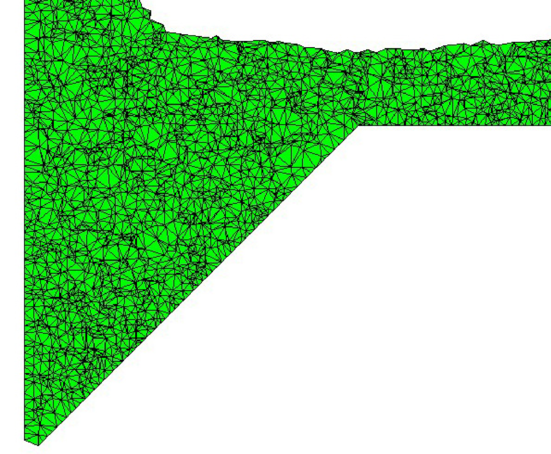(g) $t=3.0$s (h) $t=4.0$s Figure 9: Positions of the fluid domain at different time steps.

This simple example is interesting to show the quality of the boundary identification procedure. Another aim is to evaluate the volume variation from the incompressibility point of view, as well as the preservation of the total volume of the fluid due to possible errors in the boundary recognition using the Alpha-Shape method. Figure 10 shows the total fluid volume during the different time steps for the three different meshes. The charge of volume is insignificant for the fine mesh and becomes larger but acceptable for the coarse meshes.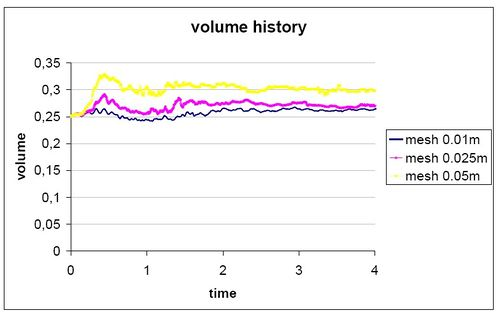Figure 10: Total volume change as a function of time for different meshes.

It must be noted that the main difference between the PFEM and the classical FEM is just the remeshing technique and the evaluation of the boundary position at each time step. The rest of the steps in the computation are coincident with those of the classical FEM. This simple example shows that in spite of the permanent remeshing and the evaluation of the boundary position via the Alpha-Shape method, the total fluid mass is preserved. We note however, that a good selection of the ${\textstyle \alpha }$ parameter is essential for the good behaviour of the boundary recognition process. Examples showing the accuracy of the PFEM for fixed boundary problems can be found in .

## 6 TREATMENT OF CONTACT CONDITIONS IN THE PFEM

### 6.1 Contact between the fluid and a fixed boundary

The motion of the solid is governed by the action of the fluid flow forces induced by the pressure and the viscous stresses acting at the common boundary ${\textstyle \Gamma _{FS}}$, as mentioned above.

The condition of prescribed velocities at the fixed boundaries in the PFEM are applied in strong form to the boundary nodes. These nodes might belong to fixed external boundaries or to moving boundaries linked to the interacting solids. Contact between the fluid particles and the fixed boundaries is accounted for by the incompressibility condition which naturally prevents the fluid nodes to penetrate into the solid boundaries (Figure 11). This simple way to treat the fluid-wall contact at mesh generation level is a distinct and attractive feature of the PFEM formulation.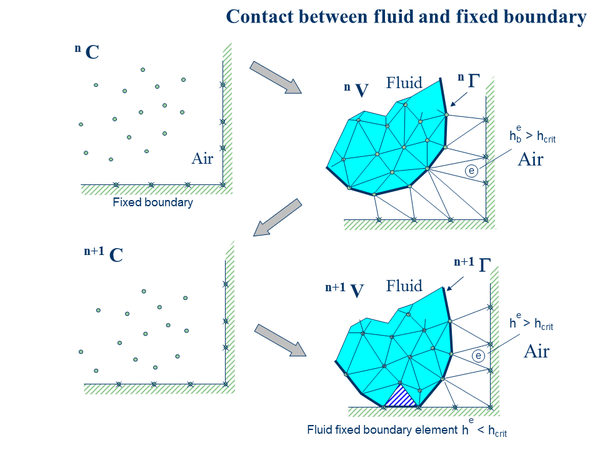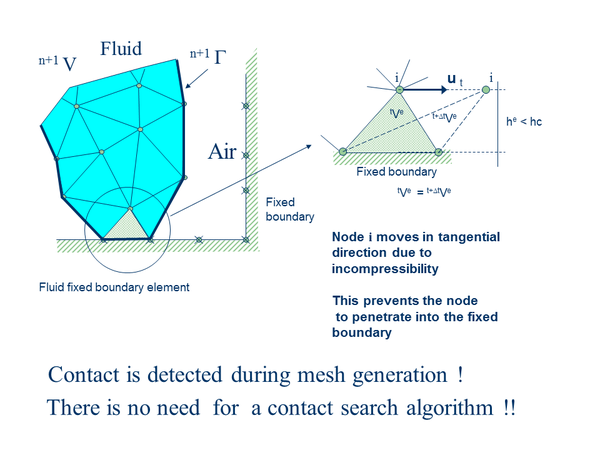Figure 11: Automatic treatment of contact conditions at the fluid-wall interface

### 6.2 Contact between solid-solid interfaces

The contact between two solid interfaces is simply treated by introducing a layer of contact elements between the two interacting solid interfaces. This layer is automatically created during the mesh generation step by prescribing a minimum distance (${\textstyle h_{c}}$) between two solid boundaries. If the distance exceeds the minimum value (${\textstyle h_{c}}$) then the generated elements are treated as fluid elements. Otherwise the elements are treated as contact elements where a relationship between the tangential and normal forces and the corresponding displacement is introduced so as to model elastic and frictional contact effects in the normal and tangential directions, respectively (Figure 12).

This algorithm has proven to be very effective and it allows to identifying and modeling complex frictional contact conditions between two or more interacting bodies moving in water in an extremely simple manner. Of course the accuracy of this contact model depends on the critical distance above mentioned.

This contact algorithm can also be used effectively to model frictional contact conditions between rigid or elastic solids in standard structural mechanics applications. Figures 1316 show examples of application of the contact algorithm to the bumping of a ball falling in a container, the failure of a domino set, the failure of an arch formed by a collection of stone blocks under a seismic loading and the motion of five tetrapods as they fall and slip over an inclined plane, respectively. The images in Figures 13 and 17 show explicitely the layer of contact elements which controls the accuracy of the contact algorithm.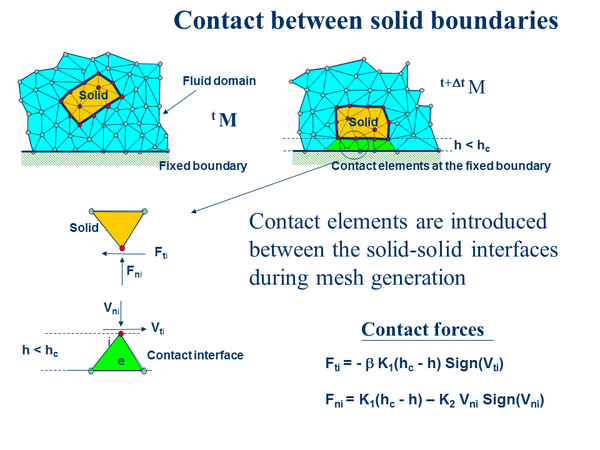Figure 12: Contact conditions at a solid-solid interface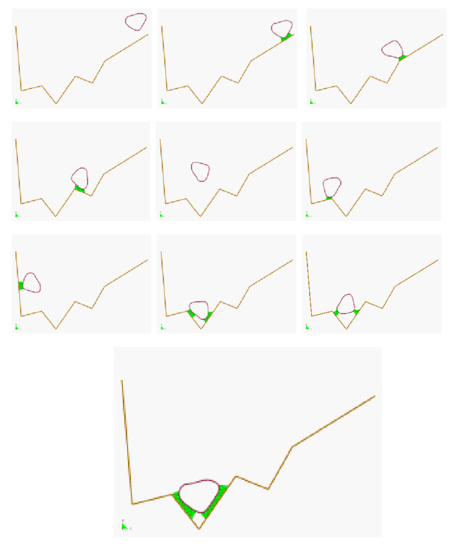Figure 13: Bumping of a ball within a container. The layer of contact elements is shown at each contact instant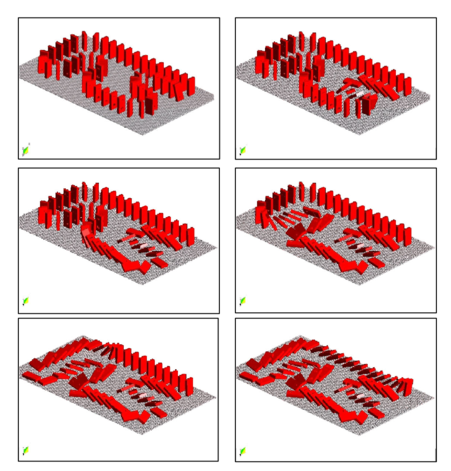Figure 14: Failure of a domino set. The distance between the domino chips shows the contact element layer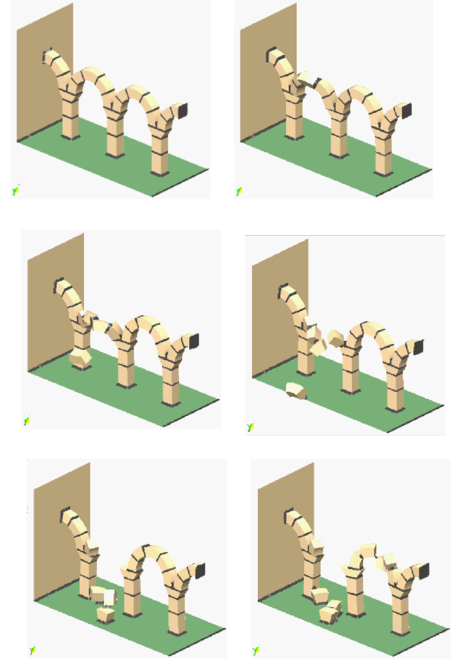Figure 15: Failure of an arch formed by stone blocks under seismic loading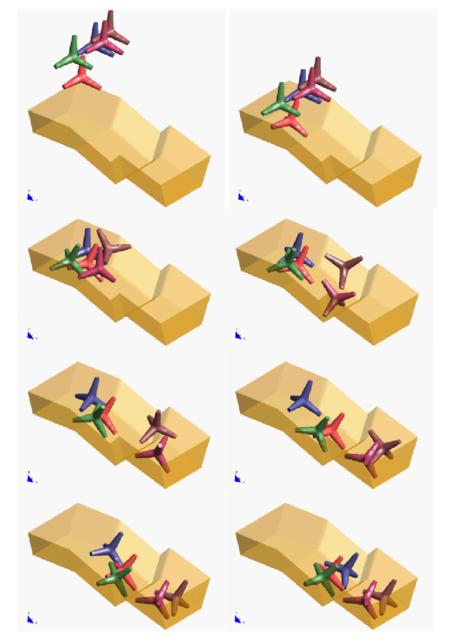Figure 16: Motion of five tetrapods on an inclined plane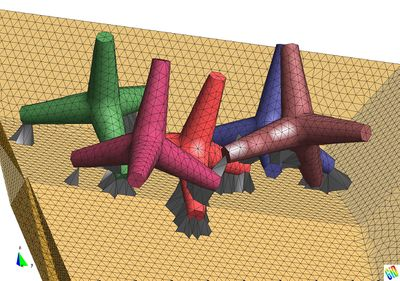Figure 17: Detail of five tetrapods on an inclined plane. The layer of elements modeling the frictional contact conditions is shown

## 7 MODELING OF BED EROSION

Prediction of bed erosion and sediment transport in open channel flows are important tasks in many areas of river and environmental engineering. Bed erosion can lead to instabilities of the river basin slopes. It can also undermine the foundation of bridge piles thereby favouring structural failure. Modeling of bed erosion is also relevant for predicting the evolution of surface material dragged in earth dams in overspill situations. Bed erosion is one of the main causes of environmental damage in floods.

Bed erosion models are traditionally based on a relationship between the rate of erosion and the shear stress level [14,33]. The effect of water velocity on soil erosion was studied in . In a recent work we have proposed an extension of the PFEM to model bed erosion . The erosion model is based on the frictional work at the bed surface originated by the shear stresses in the fluid. The resulting erosion model resembles Archard law typically used for modeling abrasive wear in surfaces under frictional contact conditions [1,22].

The algorithm for modeling the erosion of soil/rock particles at the fluid bed is the following:

1. Compute at every point of the bed surface the resultant tangential stress ${\textstyle \tau }$ induced by the fluid motion. In 3D problems ${\textstyle \tau =(\tau _{s}^{2}+\tau _{t})^{2}}$ where ${\textstyle \tau _{s}}$ and ${\textstyle \tau _{t}}$ are the tangential stresses in the plane defined by the normal direction ${\textstyle {n}}$ at the bed node. The value of ${\textstyle \tau }$ for 2D problems can be estimated as follows:
 $\tau _{t}=\mu \gamma _{t}$
(34.a)
2. with

 $\gamma _{t}={1 \over 2}{\partial v_{t} \over \partial n}={v_{t}^{k} \over 2h_{k}}$
(34.b)

where ${\textstyle v_{t}^{k}}$ is the modulus of the tangential velocity at the node ${\textstyle k}$ and ${\textstyle h_{k}}$ is a prescribed distance along the normal of the bed node ${\textstyle k}$. Typically ${\textstyle h_{k}}$ is of the order of magnitude of the smallest fluid element adjacent to node ${\textstyle k}$ (Figure 18).

3. Compute the frictional work originated by the tangential stresses at the bed surface as
 $W_{f}=\int _{\circ }^{t}\tau _{t}\gamma _{t}\,dt=\int _{\circ }^{t}{\mu \over 4}\left({v_{t}^{k} \over h_{k}}\right)^{2}dt$
(35)
4. Eq.(35) is integrated in time using a simple scheme as

 ${}^{n}W_{f}={}^{n-1}W_{f}+\tau _{t}\gamma _{t}\,\Delta t$
(36)
5. The onset of erosion at a bed point occurs when ${\textstyle {}^{n}W_{f}}$ exceeds a critical threshold value ${\textstyle W_{c}}$ defined empirically according to the specific properties of the bed material.
6. If ${\textstyle {}^{n}W_{f}>W_{c}}$ at a bed node, then the node is detached from the bed region and it is allowed to move with the fluid flow, i.e. it becomes a fluid node. As a consequence, the mass of the patch of bed elements surrounding the bed node vanishes in the bed domain and it is transferred to the new fluid node. This mass is subsequently transported with the fluid. Conservation of mass of the bed particles within the fluid is guaranteed by changing the density of the new fluid node so that the mass of the suspended sediment traveling with the fluid equals the mass originally assigned to the bed node. Recall that the mass assigned to a node is computed by multiplying the node density by the tributary domain of the node.
7. Sediment deposition can be modeled by an inverse process to that described in the previous step. Hence, a suspended node adjacent to the bed surface with a velocity below a threshold value is assigned to the bed surface. This automatically leads to the generation of new bed elements adjacent to the boundary of the bed region. The original mass of the bed region is recovered by adjusting the density of the newly generated bed elements.

Figure 18 shows an schematic view of the bed erosion algorithm proposed.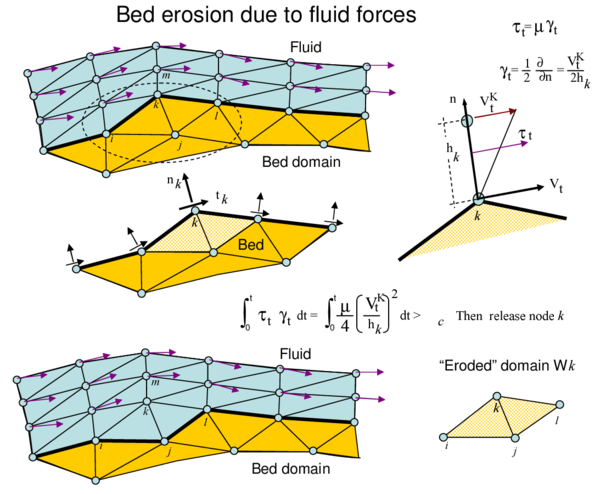Figure 18: Modeling of bed erosion by dragging of bed material

## 8 FSI EXAMPLES

The examples chosen show the applicability of the PFEM to solve problems involving large motions of the free surface, fluid-multibody interactions and bed erosion.

### 8.1 Rigid objects falling into water

The analysis of the motion of submerged or floating objects in water is of great interest in many areas of harbour and coastal engineering and naval architecture among others.

Figure 19 shows the penetration and evolution of a cube and a cylinder of rigid shape in a container with water. The colours denote the different sizes of the elements at several times. In order to increase the accuracy of the FSI problem smaller size elements have been generated in the vicinity of the moving bodies during their motion (Figure 20).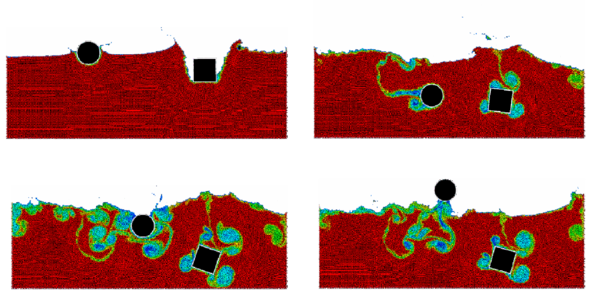Figure 19: 2D simulation of the penetration and evolution of a cube and a cylinder in a water container. The colours denote the different sizes of the elements at several times.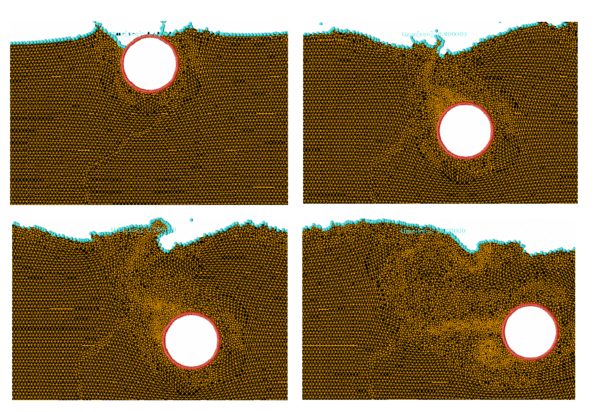Figure 20: Detail of element sizes during the motion of a rigid cylinder within a water container.

### 8.2 Impact of water streams on rigid structures

Figure 21 shows an example of a wave breaking within a prismatic container including a vertical cylinder. Figure 22 shows the impact of a wave on a vertical column sustained by four pillars. The objective of this example was to model the impact of a water stream on a bridge pier accounting for the foundation effects.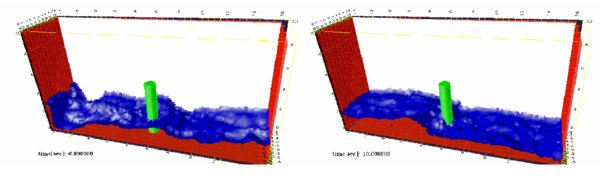Figure 21: Evolution of a water column within a prismatic container including a vertical cylinder.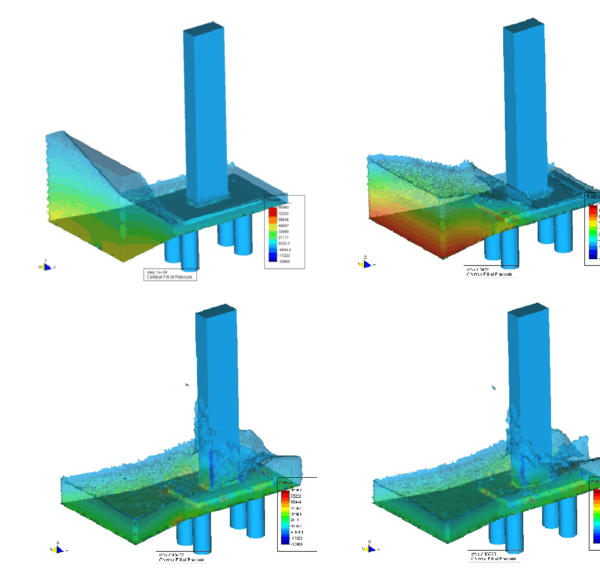Figure 22: Impact of a wave on a prismatic column on a slab sustained by four pillars.

### 8.3 Dragging of objects by water streams

Figure 23 shows the effect of a wave impacting on a rigid cube representing a vehicle. This situation is typical in flooding and Tsunami situations. Note the layer of contact elements modeling the frictional contact conditions between the cube and the bottom surface.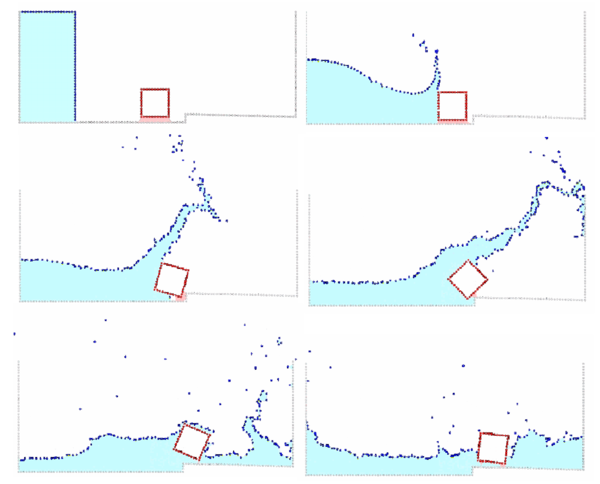Figure 23: Dragging of a cubic object by a water stream.

### 8.4 Impact of sea waves on breakwaters and piers

Figure 24 shows the 3D simulation of the impact of a wave generated in an experimental flume on a collection of rigid rocks representing a breakwater. Details of the water-rock interaction are shown in Figure 25.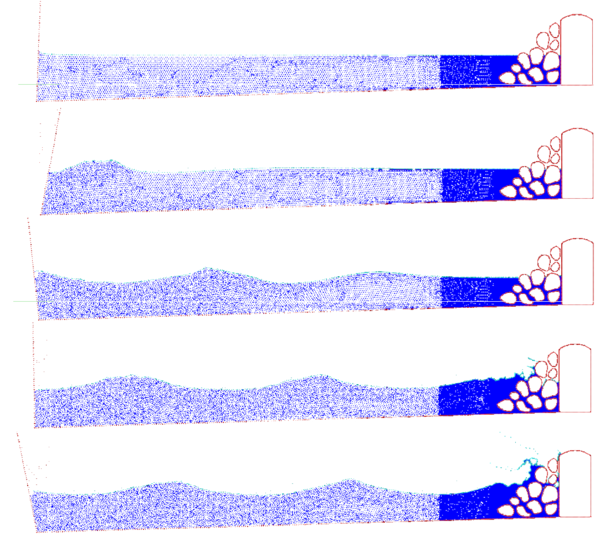Figure 24: Generation and impact of a wave on a collection of rocks in a breakwater.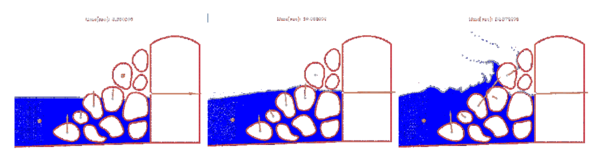Figure 25: Detail of the impact of a wave on a breakwater. The arrows indicate the water force on the rocks at different instants.

Figure 26 shows a 3D analysis of a similar problem. Figure 27 shows the 3D simulation of the interaction of a wave with a vertical pier formed by a collection of reinforced concrete cylinders.

The examples shown in Figures 28 and 29 evidence the potential of the PFEM to solve 3D problems involving complex interactions between water and moving solid objects. Figure 28 shows the simulation of the falling of two tetrapods in a water container. Figure 29 shows the motion of a collection of ten tetrapods placed in a slope under an incident wave.

Figure 30 shows a detail of the complex three-dimensional interactions between the water particles and the tetrapods and between the tetrapods themselves, which can be easily modeled with the PFEM.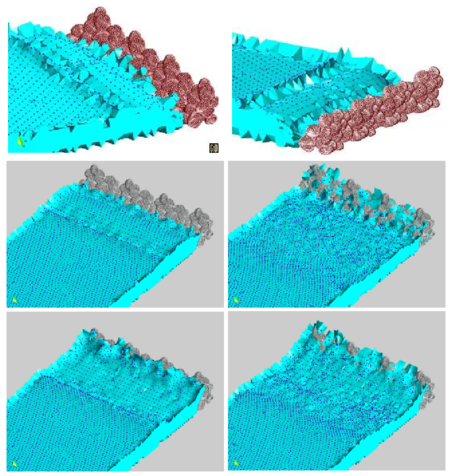Figure 26: 3D simulation of the impact of a wave on a collection of rocks in a breakwater.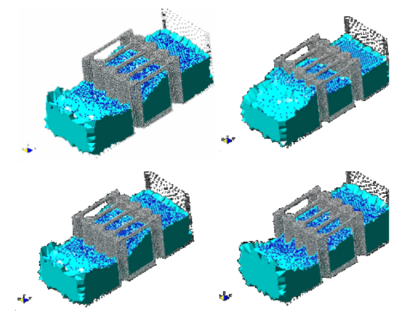Figure 27: Interaction of a wave with a vertical pier formed by reinforced concrete cylinders.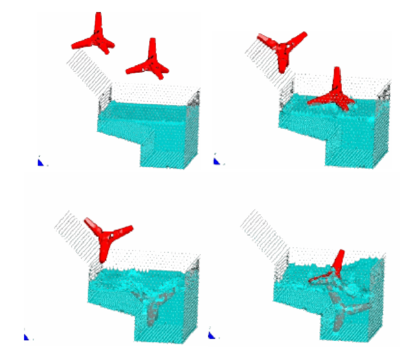Figure 28: Motion of two tetrapods falling in a water container.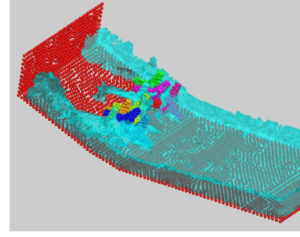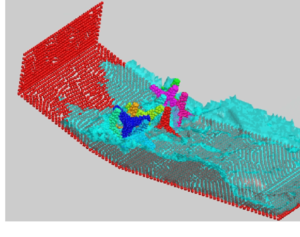Figure 30: Detail of the motion of ten tetrapods on a slope under an incident wave. The figure shows the complex interactions between the water particles and the tetrapods.

### 8.5 Erosion of a 3D earth dam due to an overspill stream

We present finally a simple, schematic, but very illustrative example showing the potential of the PFEM to model bed erosion in free surface flows.

The example represents the erosion of an earth dam under a water stream running over the dam top. A schematic geometry of the dam has been chosen to simplify the computations. Sediment deposition is not considered in the solution. The images of Figure 31 show the progressive erosion of the dam until the whole dam is dragged out by the fluid flow.

Other applications of the PFEM to bed erosion problems can be found in .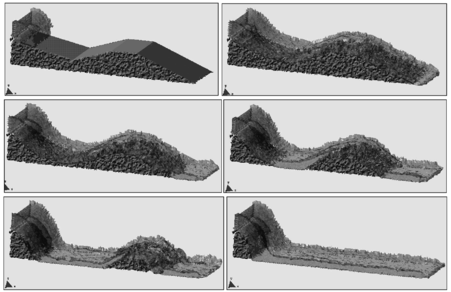Figure 31: Erosion of a 3D earth dam due to an overspill stream.

## 9 CONCLUSIONS

The particle finite element method (PFEM) is ideal to treat problems involving fluids with free surfaces and submerged or floating structures and bodies within a unified Lagrangian finite element framework. Problems such as fluid-structure interaction, large motion of fluid or solid particles, surface waves, water splashing, separation of water drops, frictional contact situations between fluid-solid and solid-solid interfaces, bed erosion, etc. can be easily solved with the PFEM. The success of the method lies in the accurate and efficient solution of the equations of an incompressible fluid and of solid dynamics using an updated Lagrangian formulation and a stabilized finite element method, allowing the use of low order elements with equal order interpolation for all the variables. Other essential solution ingredients are the efficient regeneration of the finite element mesh using an extended Delaunay tesselation, the identification of the boundary nodes using an Alpha-Shape type technique and the simple algorithm to treat frictional contact conditions at fluid-solid and solid-solid interfaces via mesh generation. The examples presented have shown the great potential of the PFEM for solving a wide class of practical FSI problems in engineering. Examples of validation of the PFEM results with data from experimental tests are reported in .

## ACKNOWLEDGEMENTS

Thanks are given to Mrs. M. de Mier for many useful suggestions. This research was partially supported by project SEDUREC of the Consolider Programme of the Ministerio de Educación y Ciencia of Spain.

### Document informationPublished on 01/01/2008

DOI: 10.1016/j.cma.2007.06.005

### Document Score0

Times cited: 121
Views 225
Recommendations 0